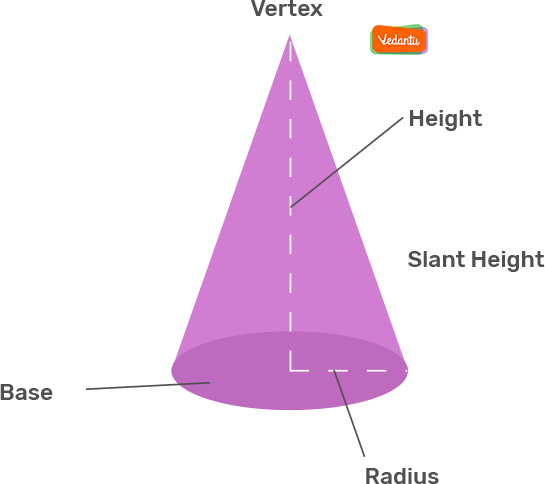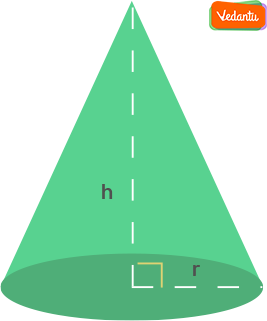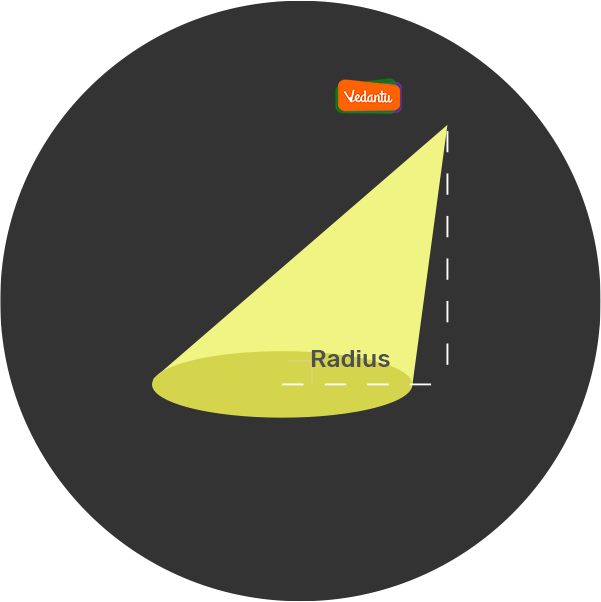# Cone Formula## What is Cone?

• A cone is a three-dimensional shape in geometry that narrows smoothly from a flat base (usually circular base) to a point(which forms an axis to the centre of the base) called the apex or vertex.

• We can also define the cone as a pyramid that has a circular cross-section, unlike a pyramid that has a triangular cross-section. These cones are also known as circular cones.## Types of Cone

As we have already discussed a brief definition of the cone, now let’s talk about its types now. Basically, there are two types of cones:

1. ### Right Circular Cone1. ### Oblique Cone## Cone Definition

Cone is a three-dimensional structure having a circular base where a set of line segments connect all of the points on the base to a common point called the apex.

There is a predefined set of formulas for the calculation of curved surface area as well as the total surface area of a cone, which is collectively known as the cone formula.

The curved surface area of a cone formula = πrl

The total surface area of a cone formula = πr(l+r)

$l = \sqrt{h^{2} + r^{2}}$

Where r equals the base radius, h equals the height, and l equals the slant height of the cone.

## Cone Formulas

1. ### Area of the Circular Base (Base Area):

The base is a simple circle and the area of a circle is given as:

Area of the base of circle = πr²

⇒ Where r is equal to the base radius of the cone.

1. ### Area of the Curved Surface:

The circumference of the base of the cone equals 2πr.

Therefore, the area of the curved surface = ½ × l × 2πr

Area of the curved surface = πrl

Total Surface Area of a Cone Formula = Area of the circular base + Area of the curved surface

⇒ Total Surface Area of a Cone Formula= πr²+πrl

⇒ Total surface area of a cone Formula = πr (l + r)

Note - The formula for the surface area and volume of the cone is derived here based on its height(h), radius(r), and slant height(l).

1. ### Slant Height

The slant height of a right circular cone is the distance from the vertex or apex to the point on the outer line of the circular base of the cone. The formula for slant height can be derived easily by the Pythagoras Theorem.

Slant Height, $l = \sqrt{h^{2} + r^{2}}$

1. ### Volume of the Cone

We can write the volume of the cone(V) with a radius of its circular base as “r”, height from the vertex to the base as “h”, and length of the edge of the cone is “l”.

Volume(V) = ⅓ πr²h cubic units.

1. ### Surface Area of the Cone

The surface area of a right circular cone is equal to the sum of its lateral surface area(πrl)and surface area of the circular base(πr²). Therefore,

The total surface area of the cone = πrl + πr² or  Area = πr(l + r)

We can put the value of slant height and we can calculate the area of the cone.

Book your Free Demo session
Get a flavour of LIVE classes here at VedantuQuestion 1. What is the CSA and TSA of Cones?

Answer. It is equal to the sum of the curved surface and the base of the cone. The formula to calculate the total surface area of a cone is given by Total Surface area of a cone formula(TSA) = CSA + Area of Circular Base. TSA = πr(r + l).

Question 2. What is the Slant Height of a Cone?

Answer. The slant height is the shortest possible distance from the base to the apex along the surface of the solid. It is usually denoted either s or l. For example, in a right circular cone, if we pick a point on the edge of the base and then connect it to the apex with a straight segment, we will have our slant height.

Question 3. Are LSA and CSA the Same?

Answer. TSA or total surface area is the sum of areas of all the surfaces of an object. LSA or lateral surface area is the area of the lateral surfaces of any object. CSA or curved surface area is equal to the area of the curved surfaces of an object. The CSA of the cylinder or hemisphere is the same as their LSA.

Comment